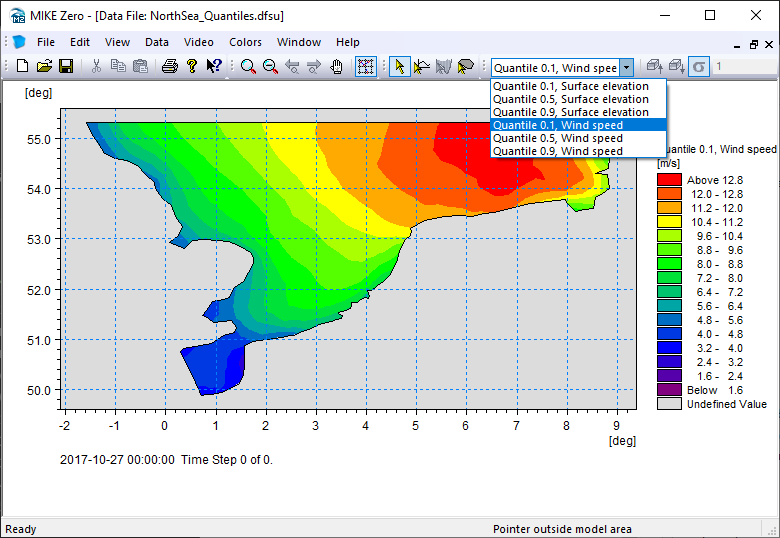# Statistics#

After running your MIKE simulation, you would often want to make different kinds of summary statistics of your data - both for your own understanding and for communicating your results.

Examples of statistics

• Min, max, mean, standard deviation

• Quantiles/percentiles (e.g. median, interquartile range, return period etc)

• Probability/frequency of exceedance

Types of aggregations

• Total - aggregate all data to a single number

• Temporal - aggregate all time steps; for a dfsu 2d, the result would be a map

• Spatial - aggregate all elements to a single value per time step

• Others: monthly, by layer, spatial bin, sub domain etc…

Ways of calculating

• mikeio.generic (temporal aggregations only; larger-than-memory)

• custom code (typically with NumPy)

import mikeio
import mikeio.generic as generic


## Dataset / DataArray#

For smaller dfs files (maybe up to 2GB) it can be convenient to read the data to memory before doing aggregations. The MIKEIO.Dataset class have several methods for aggregating data along an axis. See the generic section below for larger-than-memory data.

ds = mikeio.read("data/NorthSea_HD_and_windspeed.dfsu")
ds

<mikeio.Dataset>
dims: (time:67, element:958)
time: 2017-10-27 00:00:00 - 2017-10-29 18:00:00 (67 records)
geometry: Dfsu2D (958 elements, 570 nodes)
items:
0:  Surface elevation <Surface Elevation> (meter)
1:  Wind speed <Wind speed> (meter per sec)


### Temporal aggregations: mean#

The default is to aggregate along the time axis - the output will therefore be a map.

dsm  = ds.mean()
mean_ws = dsm["Wind speed"]
mean_ws.shape

(958,)

mean_ws.plot(title="Mean wind speed");### Spatial aggregations#

The Dataset aggregation methods (e.g. mean) takes an axis argument. If we give it the spatial axis (or the string ‘space’), it will produce a time series of spatially aggregated values.

Note

It’s important to note that the spatial aggregations here ignores element areas! Only average takes a weights argument.

ds.mean(axis="space").plot();Dataset/DataArray has other methods for calculating typical statistics, e.g. max, quantile…

ds.Wind_speed.max(axis="space").plot(title="Max wind speed");ds.Wind_speed.quantile(q=[0.1,0.5,0.9],axis="space").plot();It’s important to know that the element area is not taking into account when doing the spatial aggregations! Only Dataset.average supports weighted averages.

df = ds[["Wind speed"]].mean(axis="space").to_dataframe().rename(columns={"Wind speed":"Simple mean"})

area=ds.geometry.get_element_area()
df['Weighted'] = ds[["Wind speed"]].average(axis="space", weights=area).to_dataframe()

df.plot(title="Mean wind speed (simple vs weighted by element area)");ds

<mikeio.Dataset>
dims: (time:67, element:958)
time: 2017-10-27 00:00:00 - 2017-10-29 18:00:00 (67 records)
geometry: Dfsu2D (958 elements, 570 nodes)
items:
0:  Surface elevation <Surface Elevation> (meter)
1:  Wind speed <Wind speed> (meter per sec)


Inline exercise

1. Create a new DataArray with the temporal mean of the Surface elevation $$\eta$$

2. Create a new DataArray with the Surface elevation anomaly, $$\eta - \overline{\eta}$$

3. Plot the last time step of the anomaly

# insert your code here


### Quantiles to file#

dsq  = ds.quantile(q=[0.1,0.5,0.9])
dsq

<mikeio.Dataset>
dims: (element:958)
time: 2017-10-27 00:00:00 (time-invariant)
geometry: Dfsu2D (958 elements, 570 nodes)
items:
0:  Quantile 0.1, Surface elevation <Surface Elevation> (meter)
1:  Quantile 0.5, Surface elevation <Surface Elevation> (meter)
2:  Quantile 0.9, Surface elevation <Surface Elevation> (meter)
3:  Quantile 0.1, Wind speed <Wind speed> (meter per sec)
4:  Quantile 0.5, Wind speed <Wind speed> (meter per sec)
5:  Quantile 0.9, Wind speed <Wind speed> (meter per sec)


Write to a new dfsu file

dsq.to_dfs("NorthSea_Quantiles.dfsu")### Total#

Aggregating over all data (both time and space) can be done from the Dataset in a few ways:

• ds.describe() - will give you summary statistics like pandas df.describe()

• using axis=None in ds.mean(), ds.min()

• using standard NumPy aggregation functions on the Dataset data e.g. ds[“Wind speed”].mean()

ds.describe()

Surface elevation Wind speed
count 64186.000000 64186.000000
mean 0.449857 12.772705
std 0.651157 3.694293
min -2.347003 1.190171
25% 0.057831 10.376003
50% 0.466257 12.653086
75% 0.849586 14.885848
max 3.756879 26.213045
ds.min(axis=None).to_dataframe()

Surface elevation Wind speed
2017-10-27 -2.347003 1.190171
ds["Wind speed"].values.min()

1.1901706


## Generic#

The MIKEIO.generic submodule can produce common temporal statistics on any dfs file (of any size). The output will be a new dfs file. Currently, generic has these methods for calculating statistics:

• avg_time()

• quantile()

generic.avg_time("data/NorthSea_HD_and_windspeed.dfsu", "NorthSea_avg.dfsu")

ds = mikeio.read("NorthSea_avg.dfsu", items="Wind speed")
ds

<mikeio.Dataset>
dims: (time:1, element:958)
time: 2017-10-27 00:00:00 (time-invariant)
geometry: Dfsu2D (958 elements, 570 nodes)
items:
0:  Wind speed <Wind speed> (meter per sec)

ds["Wind speed"].plot(title="Mean wind speed");generic.quantile("data/NorthSea_HD_and_windspeed.dfsu", "NorthSea_Quantiles2.dfsu", q=[0.1, 0.5, 0.9])## Custom#

ds = mikeio.read("data/NorthSea_HD_and_windspeed.dfsu")


### Dataset.aggregate#

With aggregate we can get Dataset statistics with our “own” function, e.g. standard deviation:

import numpy as np
dsa = ds.aggregate(func=np.std)

dsa["Wind speed"].plot(label="Std [m/s]");### Exceedance probability#

Let’s find out how often the wind exceeds 12m/s in our simulation.

import matplotlib.pyplot as plt

nt = ds.n_timesteps
one_to_zero = 1. - np.arange(1., nt + 1.)/nt

val = ds["Wind speed"].isel(element=0).values
plt.plot(ds.time, val);
plt.plot(ds.time[val>12], val[val>12],'.r');
plt.axhline(y=12,color='r')
plt.ylabel('Wind speed [m/s]')
plt.title('How often is the wind speed above 12m/s (element 0)?');plt.plot(np.sort(val), one_to_zero);
plt.xlabel('Wind speed [m/s]')
plt.ylabel('Probability of exceedance [-]')
plt.axvline(x=12,color='r')
plt.title('Wind speed exceedance in element 0');# Create empty DataArray
item=mikeio.ItemInfo(mikeio.EUMType.Probability)
data = np.full(shape=(1,ds.geometry.n_elements), fill_value=np.nan)
dae = mikeio.DataArray(data=data, time="2017-10-27", item=item, geometry=ds.geometry)

threshold = 12
for j in range(ds.n_elements):
# this is a naive and slow way of calculating this!
dat = ds["Wind speed"][:,j].values
dae[0,j] = np.interp(threshold, np.sort(dat), one_to_zero)

dae100 = dae*100
dae100.plot(title="Wind speed exceeding 12 m/s",
label="Time of Exceedance [%]", cmap="YlOrRd");total_hours = (ds.time[-1]-ds.time).total_seconds()/3600
dae_hours = dae*total_hours
dae_hours.plot(title="Wind speed exceeding 12 m/s",
label="Exceedance [Hours]", cmap="YlOrRd");